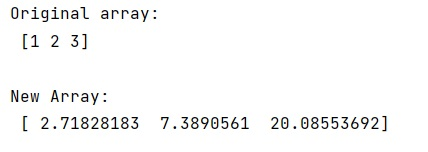# What exactly does numpy.exp() do?

Learn, what exactly does numpy.exp() do in Python?
Submitted by Pranit Sharma, on January 05, 2023

NumPy is an abbreviated form of Numerical Python. It is used for different types of scientific operations in python. Numpy is a vast library in python which is used for almost every kind of scientific or mathematical operation. It is itself an array which is a collection of various methods and functions for processing the arrays.

## numpy.exp() Method

This method of numpy is used to Calculate the exponential of all elements in the input array. It takes an argument called out which is a location into which the result is stored. If provided, it must have a shape that the inputs broadcast to. If not provided or None, a freshly-allocated array is returned. A tuple (possible only as a keyword argument) must have a length equal to the number of outputs.

It also takes an argument called where which takes a condition that is broadcast over the input. At locations where the condition is True, the out array will be set to the ufunc result. Elsewhere, the out array will retain its original value. Note that if an uninitialized out array is created via the default out=None, locations within it where the condition is False will remain uninitialized.

Let us understand with the help of an example,

## Python code to demonstrate the example of numpy.exp() method

```# Import numpy
import numpy as np

# Creating a numpy array
arr = np.array([1,2,3])

# Display original array
print("Original array:\n",arr,"\n")

# Defining an array for finding exponents
a = [1,2,3]

# Using exp function
res = np.exp(arr)

# Display result
print("New Array:\n",res)
```

Output: